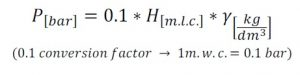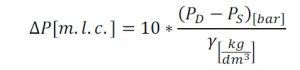The pump selection of a pump is driven by the following main parameters:
– Flow rate (Q)
– Fluid characteristics (ρ, γ, T ….)
Sometimes head can be confused with pressure during pump choice. As a matter of fact there is a strict relation between them which is defined by the fluid specific gravity, so the relation is fluid dependent. So what is the difference between pressure and head?

#### Definition of head and pressure

Head is the height given by the pump to the fluid and it is measured in meters of liquid column [m.l.c.] or simply indicated in meters [m]. The given head is fluid independent: different fluids
with different specific gravities are all lifted at the same height.
Pressure, instead, is fluid dependent and it is affected by the liquid density. In fact the force of a fixed height liquid column over a unitary area will change with different specific gravities. So in this case the same head generates different pressures.

Head is not directly measured. Manometers on the pump suction and delivery line give the measure of the pressure. Measurements given by manometers indicate differential pressures imposed by the pump between suction and discharge. These measures are read in [bar] [atm] [psi] [ft H2O] etc.. Specific gravity γ has to be considered to evaluate the correspondent head.

#### Conversions & Practical Example

As stated before, the same pump at the same working point will give always the same head with different pressures in accordance with the density γ of the working fluid.
For example, a mag drive centrifugal pump HTM 10 working at a given point Q= 7.5 m3/h H = 10 mlc operating with water and concentrated H2SO4 gives the same head (H=10m) to water ( γ= 1kg/dm3) and to sulfuric acid (γ = 1.8kg/dm3), while measurements of differential pressures between suction and delivery will be:The mathematical relationship is reported in the following equation:Also power consumption is influenced by the previous relationship of pressure, as:

#### Notes for technicians

– Previous relations are valid for low viscosity fluids (water equivalent), along with increasing viscosity, pump performances have to be reduced using pump derating rules.
– At a fixed rotational speed a centrifugal pump generates head related to the flow rate following its characteristic curve.
– The computation of the needed head that should be delivered by the pump, is not so straight as the evaluation of the desired height difference. Needed Head is composed by the following terms:

• Geodetic head: difference between suction and delivery height expressed in meters of liquid column• Difference between absolute pressure of the delivery and suction reservoir